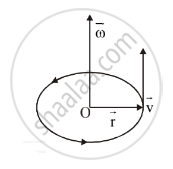# In U. C. M (Uniform Circular Motion), Prove the Relation V = W x R, Where Symbols Have Their Usual Meanings. - Physics

In U. C. M (Uniform Circular Motion), prove the relation vec v = vec w xx vec r, where symbols have their usual meanings.

#### Solution

Analytical method :
Consider a particle revolving in the anticlockwise sense along the circumference of a circle of radius r with centre O as shown.Let

vec omega= angular velocity of the particle

vec v= linear velocity of the particle

vec r= radius of the particle

In the vector form, the linear dispalcement is

vec (delta s) = vec (delta theta) times vec r

Dividing both sides by delta t we get

vec (delta s)/(delta t) = vec (delta theta)/(delta t) times vec r

lim_(delta t -> 0) vec (delta s)/(delta t) = lim_(delta t -> 0) vec (delta theta)/(delta t) times vec r

therefore vec(dS)/dt = vec(d theta)/(delta t) times vec r

but

vec(dS)/dt = vec v = Linear velocity

vec(d theta)/(delta t) = vec omega = angular velocity

therefore vec v = vec omega times vec r

Concept: Uniform Circular Motion
Is there an error in this question or solution?